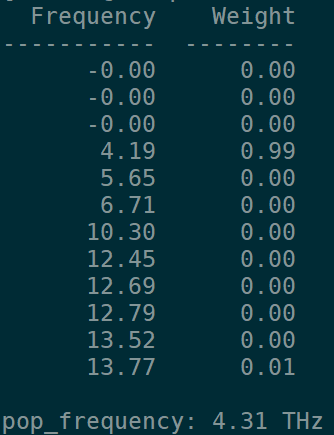# What is the difference between finite displacement(IBRION=6) and density function perturbation theory(IBRION=8) when calculate the dielectric constants， etc?

In your tutorial, the elastic and dielectric are calculated separately,
but if use the IBRION = 6 and LEPSILON = .T. , I can get both of them.
what are the differences between the differect approaches?Hi,

You would have to consult the VASP manual about the difference between IBRION = 6 and IBRION = 8. I believe one is DFPT and the other is finite differences but I am not entirely sure of the subtleties between the two approaches. See: IBRION - Vaspwiki

Naively, both approaches should be fine.

Best,
Alex

Dear Alex,